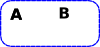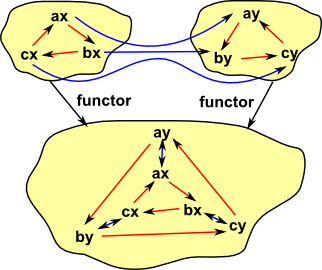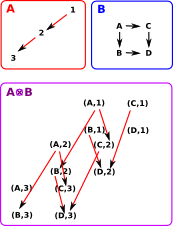# Maths - Category Theory - Compound Categories

Here we look at building new categories from existing categories:

### Opposite

There is a duality between categories by reversing the direction of the arrows.

The opposite category of C is denoted Cop

### Sub Objects

This is a generalisation of subset.

The concept of a sub-object is related to these concepts:

• Equivalence as described on page here.
• Subobject Classifier on page here.
• See page here for a non-categorical approach to sub-objects.

### Product and Sum

Product
(pullback)

More on this page

Sum
(Coproduct)
(pushout)

More on this page

universal cone over diagramgeneralisation a kind of limit a kind of colimit
set example

cartesian product{a,b,c}*{x,y}=
{{a,x},{b,x},{c,x},{a,y},{b,y},{c,y}}

disjoint union{a,b,c}+{x,y}=
{a,b,c,x,y}

group the product is given by the cartesian product with multiplication defined componentwise. free product
the free product for groups is generated by the set of all letters from a similar "almost disjoint" union where no two elements from different sets are allowed to commute.
Grp (abelian) direct sum

direct sum
consists of the elements of the direct product which have only finitely many nonzero terms (this therefore coincides exactly with the direct product, in the case of finitely many factors)

the group generated by the "almost" disjoint union (disjoint union of all nonzero elements, together with a common zero)

vector space direct sum direct sum
poset greatest lower bound
meet
least upper bound
join
base topological space   wedge
POS

greatest lower bounds (meets)

least upper bounds (joins)

Rng
Top the space whose underlying set is the cartesian product and which carries the product topology disjoint unions with their disjoint union topologies
Grf
category objects: (a,b)
morphism: (a,b)->(a',b')

tensor products are not categorical products.

In the category of pointed spaces, fundamental in homotopy theory, the coproduct is the wedge sum (which amounts to joining a collection of spaces with base points at a common base point).

#### Sum

When generating a sum for objects with structure then the structure associated with the link can be added to the sum object.#### ProductProducts for groups are discussed on this page.

## Cartesian Product

Here we extend the idea of a cartesian product of sets to other categories. The categorical product concept above defines the product by its external relationships which is the most categorical way to do it. Here we take a slightly less categorical approach and use the objects and arrows of the categories being multiplied to define their product. We treat the categories as set + structure.

The objects of the product are the same as the cartesian product of sets.

The arrows are combined in a similar way to the Cartesian and tensor products of graphs.

We can extend the concept of cartesian product of sets to categories as follows:

 Here is an ordinary cartesian product of sets.Here we have sets with structure, represented by arrows. An arrow (a, b) -> (a', b') exists in A×B if and only if either: a = a' and b->b' exists in A, or b = b' and a->a' exists in B.## Tensor Product

More about this on this page.

We can extend the concept of cartesian product of sets to categories as follows:

 The objects are the same as cartesian product of sets but the arrows are different from the cartesian example above. There is an arrow in AB only if there is an arrow in both A and B. An arrow (a, b) -> (a', b') exists in AB if and only if: b->b' exists. and a->a' exists.Looking at the case above it looks like we could recover projections to A and B from AB. However, is there an example where we can't, where one of the operands has a object with no arrows leaving it? On the right is a diagram of an extreme case where one of the operands has no arrows. In this case, by the definition given above, the tensor product will have no arrows. In this case there may be a projection from A0 to 0 (that would be the Cartesian set product) Would there be an arrow from A0 to A? maybe so, if we take a graph with no arrows as being an initial object. Perhaps this is an example which shows the cartesian product of graphs is not a product in the category theory sense?A graph with a single object and an arrow to itself is the unit: 1.## Exponential

This is a universal structure but not a limit.

## Functor Category.

We can construct a new category from an existing category 'C', where:

• The objects are the functors of 'C'.
• The morphisms are the natural transformations of 'C'.

More about Functor Category on page here.

#### Example of Functor Category

The category of all directed graphs is the functor category SetC. (see presheaves on page here)

Where C is a category with two objects connected by two morphisms

see Yoneda embedding.

## Comma/Slice Categories.

More about Comma/Slice categories on this page.

## Quotient Categories.

metadata block
see also:

Catsters youtube videos - Terminal and initial objects

Catsters youtube videos - Products and coproducts

Catsters youtube videos - Pullbacks and pushouts

Catsters youtube videos - General limits and colimits

Correspondence about this page

Book Shop - Further reading.

Where I can, I have put links to Amazon for books that are relevant to the subject, click on the appropriate country flag to get more details of the book or to buy it from them.The Princeton Companion to Mathematics - This is a big book that attempts to give a wide overview of the whole of mathematics, inevitably there are many things missing, but it gives a good insight into the history, concepts, branches, theorems and wider perspective of mathematics. It is well written and, if you are interested in maths, this is the type of book where you can open a page at random and find something interesting to read. To some extent it can be used as a reference book, although it doesn't have tables of formula for trig functions and so on, but where it is most useful is when you want to read about various topics to find out which topics are interesting and relevant to you.

Specific to this page here:

This site may have errors. Don't use for critical systems.

Copyright (c) 1998-2021 Martin John Baker - All rights reserved - privacy policy.